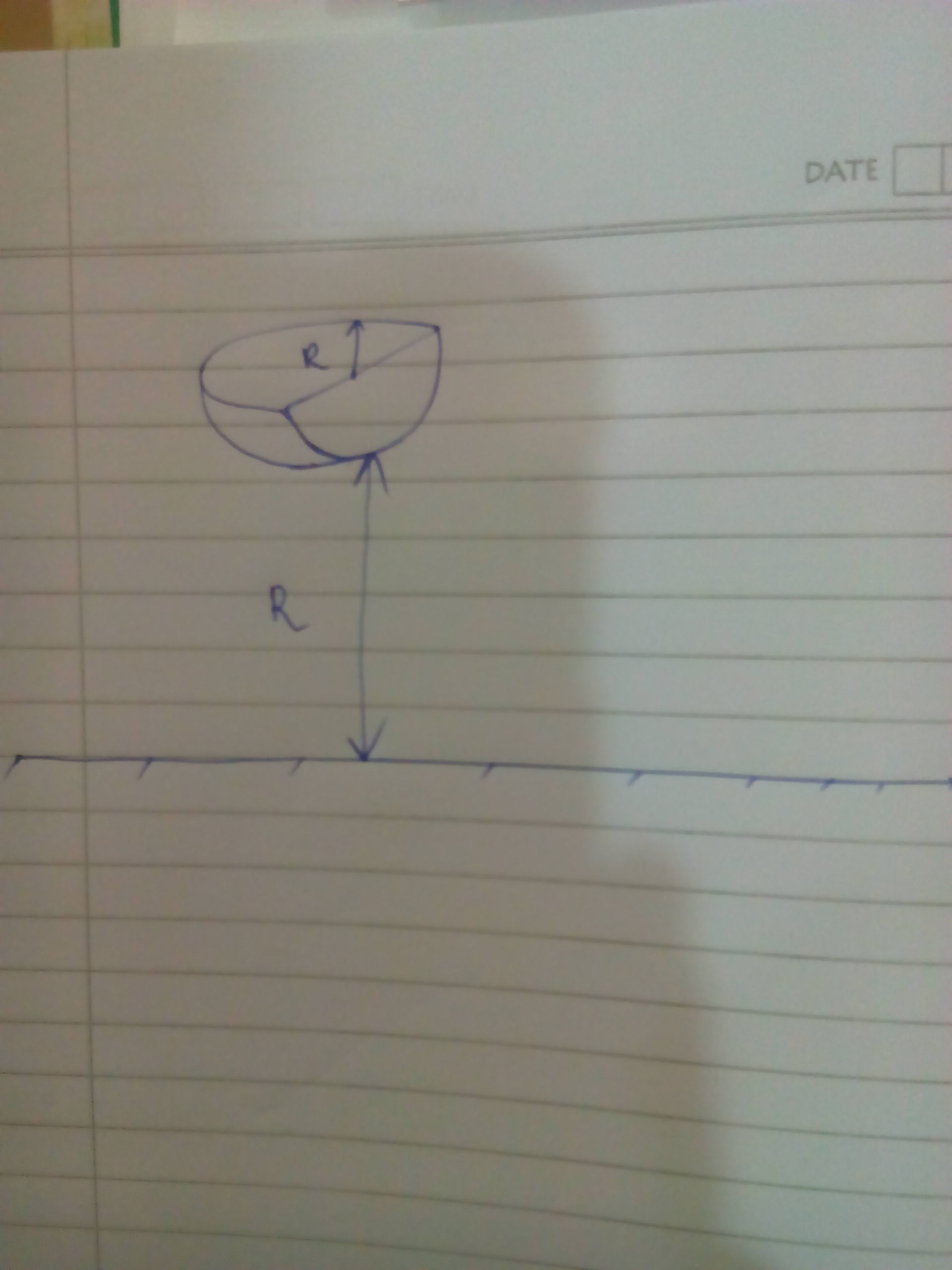# Getting back to the old things #6

A solid body of mass $M$ is in the shape of a quadrant sphere of radius $R$. It is released from rest in a position such that the curved portion is at a height $R$.

The collision is perfectly elastic. The angular velocity of the body just after striking the ground is represented in the form $\dfrac{a}{b} \sqrt{\frac{cg}{R}}$, where $g$ represents acceleration due to gravity. Here $a$ and $b$ are coprime positive integers and $c$ is square free.

Submit your answer as $a+b+c$.×

Problem Loading...

Note Loading...

Set Loading...